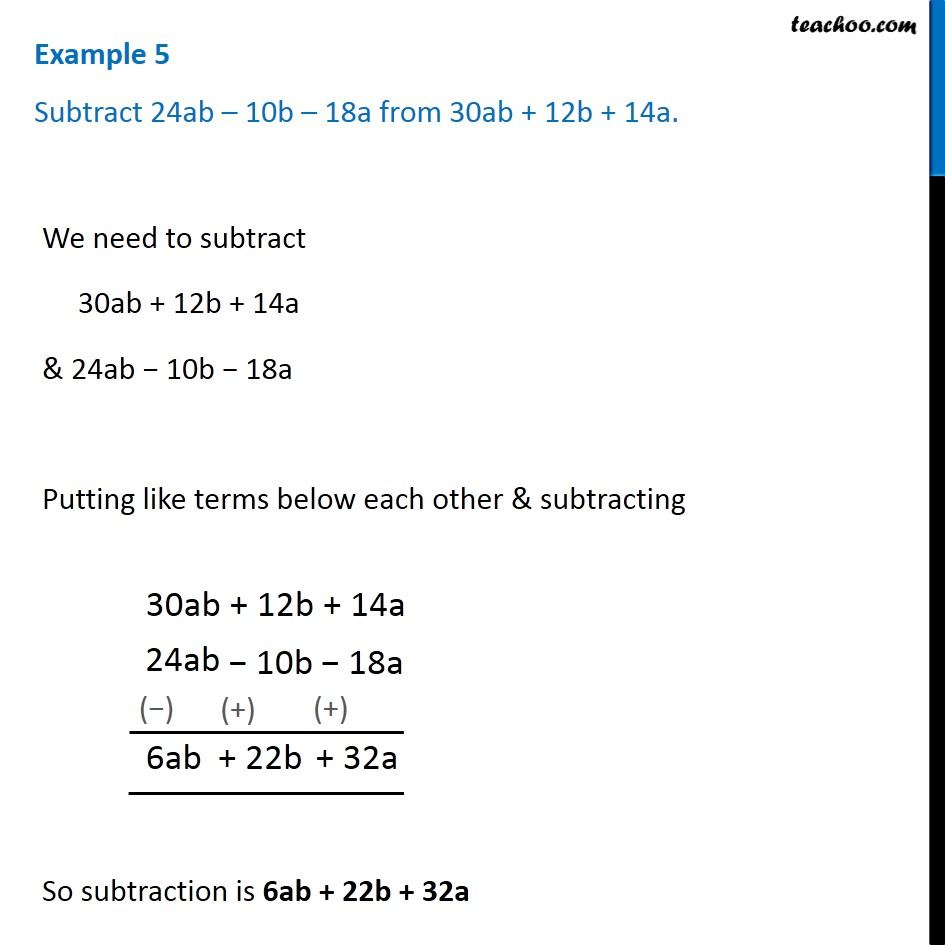Examples

Chapter 10 Class 7 Algebraic Expressions
Serial order wiseLearn in your speed, with individual attention - Teachoo Maths 1-on-1 Class

### Transcript

Question 2 Subtract 24ab – 10b – 18a from 30ab + 12b + 14a. We need to subtract 30ab + 12b + 14a & 24ab − 10b − 18a Putting like terms below each other & subtracting So subtraction is 6ab + 22b + 32a